Geometry

# Area From Rectangles to Triangles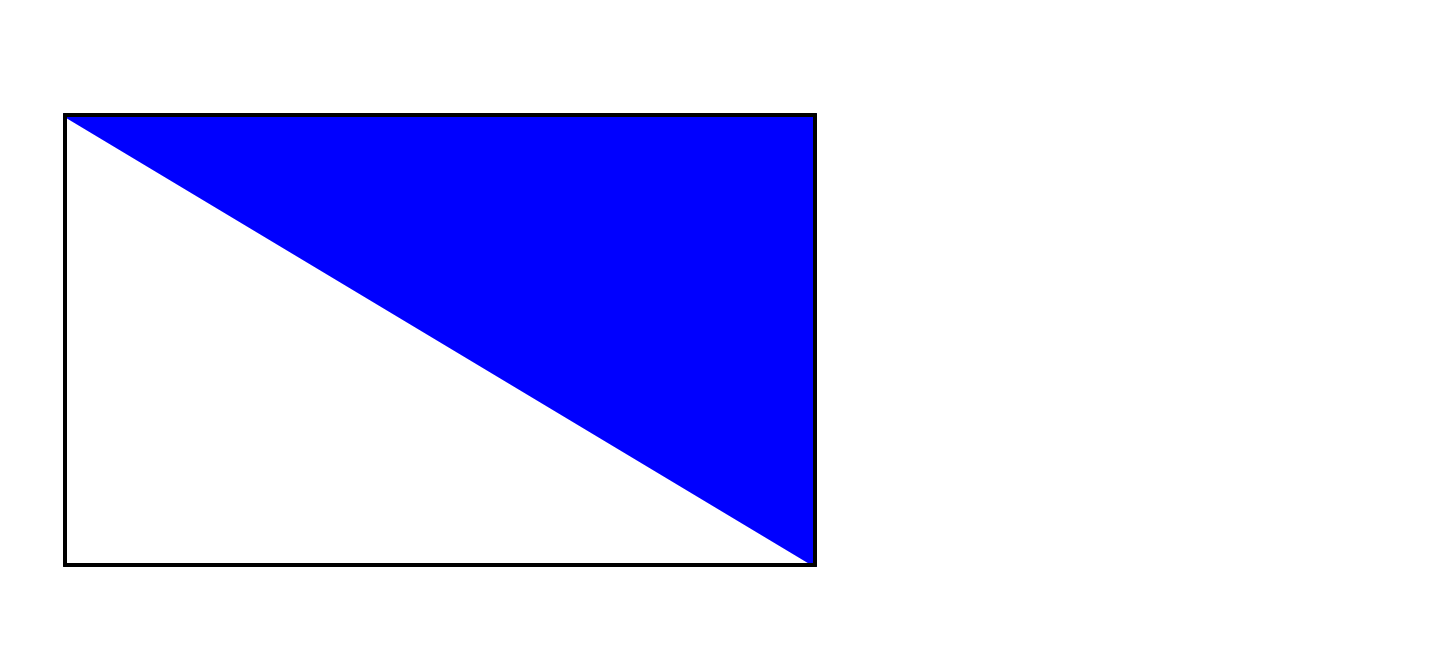The area of the rectangle is 40. What is the area of the triangle?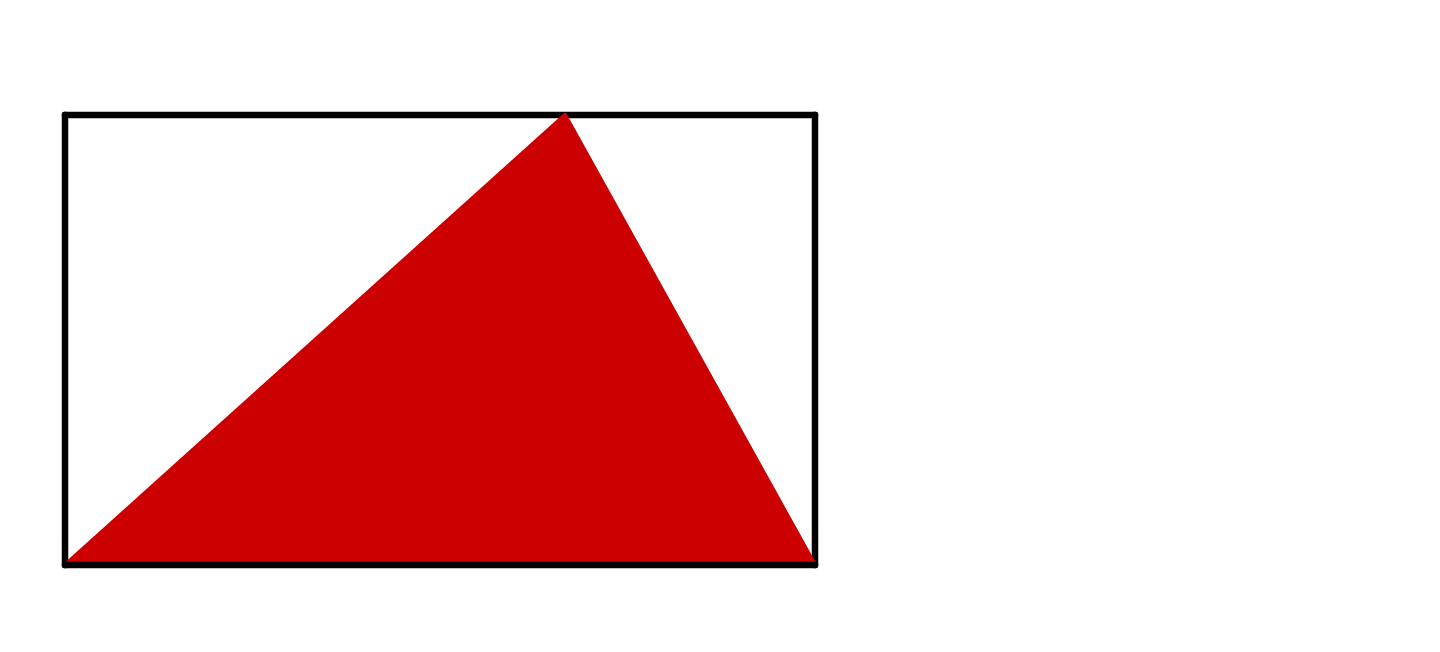The area of the rectangle is 10. What is the area of the triangle?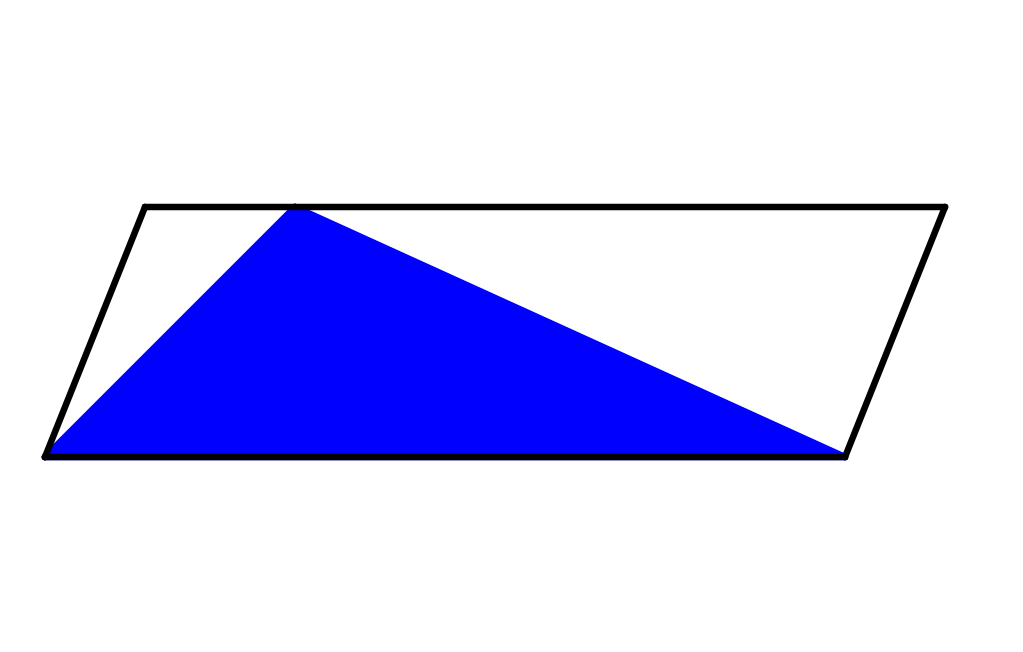The area of the parallelogram is 100. What is the area of the triangle?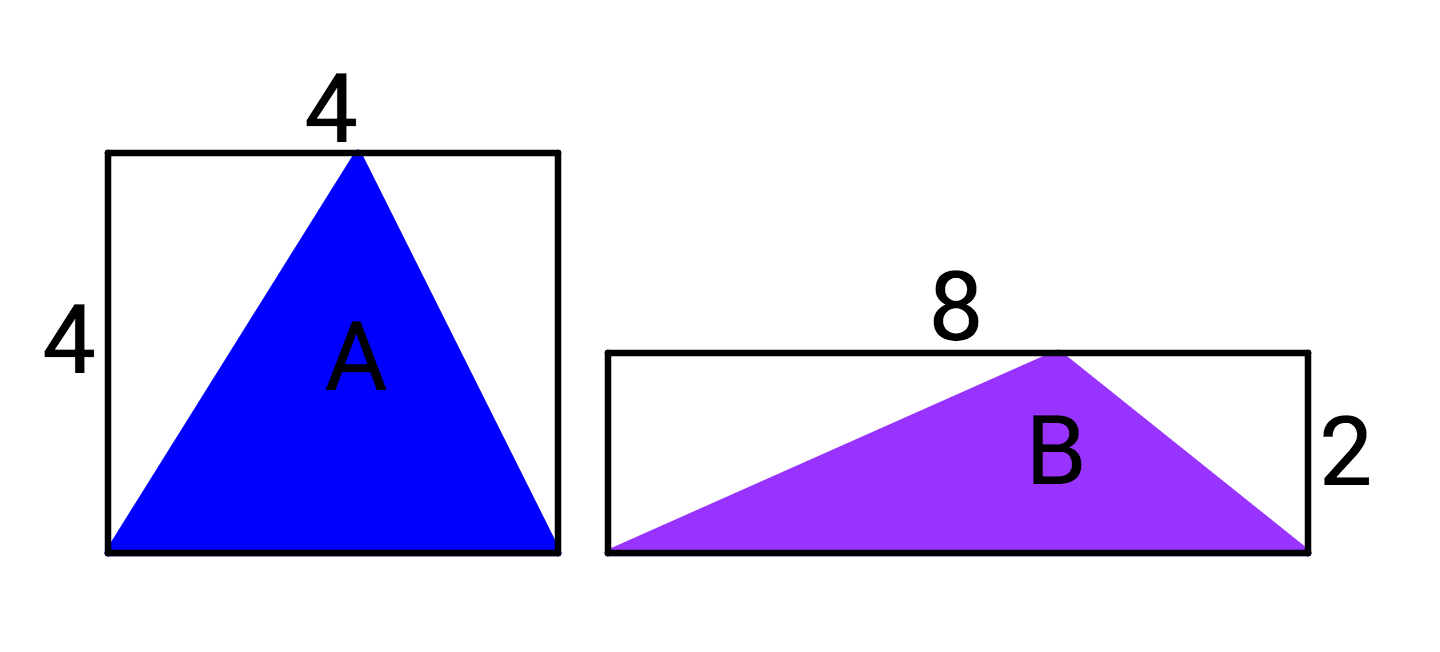Which triangle has a greater area?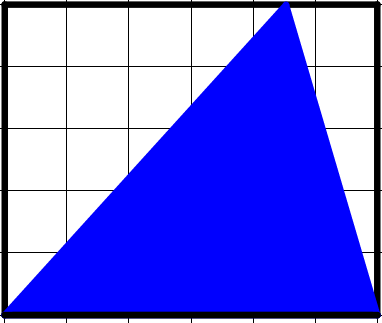If each square on the grid has an area of 1, what is the area of the triangle?

×